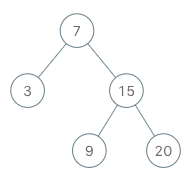# 173. Binary Search Tree Iterator

Implement the `BSTIterator` class that represents an iterator over the in-order traversal of a binary search tree (BST):

• `BSTIterator(TreeNode root)` Initializes an object of the `BSTIterator` class. The `root` of the BST is given as part of the constructor. The pointer should be initialized to a non-existent number smaller than any element in the BST.
• `boolean hasNext()` Returns `true` if there exists a number in the traversal to the right of the pointer, otherwise returns `false`.
• `int next()` Moves the pointer to the right, then returns the number at the pointer.

Notice that by initializing the pointer to a non-existent smallest number, the first call to `next()` will return the smallest element in the BST.

You may assume that `next()` calls will always be valid. That is, there will be at least a next number in the in-order traversal when `next()` is called.

Example 1:```Input
["BSTIterator", "next", "next", "hasNext", "next", "hasNext", "next", "hasNext", "next", "hasNext"]
[[[7, 3, 15, null, null, 9, 20]], [], [], [], [], [], [], [], [], []]
Output
[null, 3, 7, true, 9, true, 15, true, 20, false]

Explanation
BSTIterator bSTIterator = new BSTIterator([7, 3, 15, null, null, 9, 20]);
bSTIterator.next();    // return 3
bSTIterator.next();    // return 7
bSTIterator.hasNext(); // return True
bSTIterator.next();    // return 9
bSTIterator.hasNext(); // return True
bSTIterator.next();    // return 15
bSTIterator.hasNext(); // return True
bSTIterator.next();    // return 20
bSTIterator.hasNext(); // return False
```

Constraints:

• The number of nodes in the tree is in the range `[1, 105]`.
• `0 <= Node.val <= 106`
• At most `105` calls will be made to `hasNext`, and `next`.

• Could you implement `next()` and `hasNext()` to run in average `O(1)` time and use `O(h)` memory, where `h` is the height of the tree?

## The solution to the above question is-

/**
* Definition for a binary tree node.
* struct TreeNode {
*     int val;
*     TreeNode *left;
*     TreeNode *right;
*     TreeNode() : val(0), left(nullptr), right(nullptr) {}
*     TreeNode(int x) : val(x), left(nullptr), right(nullptr) {}
*     TreeNode(int x, TreeNode *left, TreeNode *right) : val(x), left(left), right(right) {}
* };
*/
class BSTIterator {
public:
stack<TreeNode*>st;
void inorder(TreeNode* root){

if(root==NULL)return;
st.push(root);
inorder(root->left);
}

BSTIterator(TreeNode* root) {
inorder(root);
}

int next() {
TreeNode* ans=st.top();
st.pop();

inorder(ans->right);
return ans->val;
}

bool hasNext() {
return !st.empty();
}
};

/**
* Your BSTIterator object will be instantiated and called as such:
* BSTIterator* obj = new BSTIterator(root);
* int param_1 = obj->next();
* bool param_2 = obj->hasNext();
*/